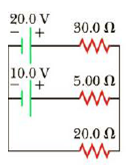Chapter 18, Problem 29P

Chapter
Section
Textbook Problem

(a) Can the circuit shown in Figure P18.29 be reduced to a single resistor connected to the batteries? Explain. (b) Find the magnitude of the current and its direction in each resistor.Figure P18.29

(a)

To determine
Whether the given circuit can be reduced to a circuit with a single resistor connected to the batteries.

Explanation

Resistors connected in parallel combination can be combined by to get equivalent resistance. But this condition is accepted when all the resistor is are connected to the same the potential difference.

Reducing the circuit cannot be made because there are three resistances R1 , R2<

(b)

To determine
The magnitude and the direction of the current in each resistor.

Still sussing out bartleby?

Check out a sample textbook solution.

See a sample solution

The Solution to Your Study Problems

Bartleby provides explanations to thousands of textbook problems written by our experts, many with advanced degrees!

Get Started